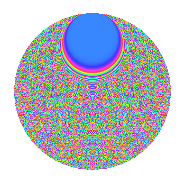# Properties

 Label 1343.2.biLevel 1343 Weight 2 Character orbit bi Rep. character $$\chi_{1343}(12,\cdot)$$ Character field $$\Q(\zeta_{208})$$ Dimension 11328 Sturm bound 240

# Related objects

## Defining parameters

 Level: $$N$$ = $$1343 = 17 \cdot 79$$ Weight: $$k$$ = $$2$$ Character orbit: $$[\chi]$$ = 1343.bi (of order $$208$$ and degree $$96$$) Character conductor: $$\operatorname{cond}(\chi)$$ = $$1343$$ Character field: $$\Q(\zeta_{208})$$ Sturm bound: $$240$$

## Dimensions

The following table gives the dimensions of various subspaces of $$M_{2}(1343, [\chi])$$.

Total New Old
Modular forms 11712 11712 0
Cusp forms 11328 11328 0
Eisenstein series 384 384 0

## Trace form

 $$11328q - 88q^{2} - 104q^{3} - 88q^{4} - 88q^{5} - 104q^{6} - 104q^{7} - 88q^{8} - 88q^{9} + O(q^{10})$$ $$11328q - 88q^{2} - 104q^{3} - 88q^{4} - 88q^{5} - 104q^{6} - 104q^{7} - 88q^{8} - 88q^{9} - 88q^{10} - 88q^{11} - 104q^{12} - 104q^{13} - 104q^{14} - 104q^{15} - 104q^{17} - 240q^{18} - 88q^{19} - 88q^{20} - 40q^{21} - 88q^{22} - 176q^{23} - 88q^{25} - 88q^{26} - 104q^{27} - 104q^{28} - 104q^{29} - 104q^{30} - 152q^{31} + 320q^{32} - 104q^{34} - 208q^{35} - 184q^{36} - 520q^{37} - 168q^{38} - 104q^{39} - 152q^{40} - 104q^{41} - 88q^{42} - 104q^{43} - 792q^{44} - 88q^{45} - 56q^{46} - 104q^{47} - 104q^{48} - 24q^{49} - 88q^{51} - 176q^{52} - 104q^{53} - 104q^{54} - 128q^{55} - 104q^{57} - 104q^{58} - 104q^{59} - 104q^{60} - 104q^{61} - 88q^{62} - 104q^{63} + 200q^{64} - 120q^{65} - 104q^{66} - 104q^{68} - 208q^{69} - 104q^{70} - 104q^{71} - 24q^{72} - 88q^{73} - 104q^{74} - 104q^{75} - 216q^{76} - 104q^{77} - 160q^{79} - 272q^{80} + 440q^{81} - 104q^{82} - 136q^{83} - 104q^{85} - 208q^{86} - 88q^{87} - 88q^{88} + 56q^{89} - 8q^{90} - 104q^{91} - 88q^{92} - 104q^{93} - 104q^{94} + 72q^{95} - 104q^{96} + 40q^{97} - 88q^{98} - 72q^{99} + O(q^{100})$$

## Decomposition of $$S_{2}^{\mathrm{new}}(1343, [\chi])$$ into newform subspaces

The newforms in this space have not yet been added to the LMFDB.

## Hecke Characteristic Polynomials

There are no characteristic polynomials of Hecke operators in the database